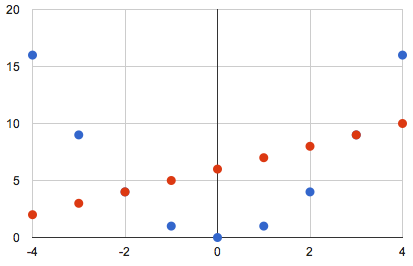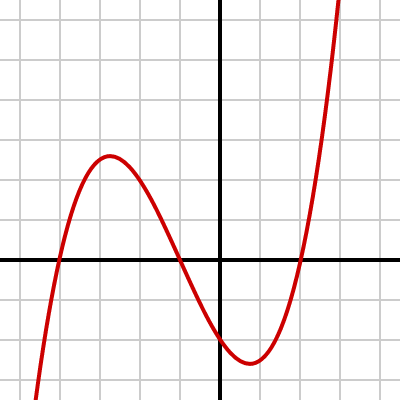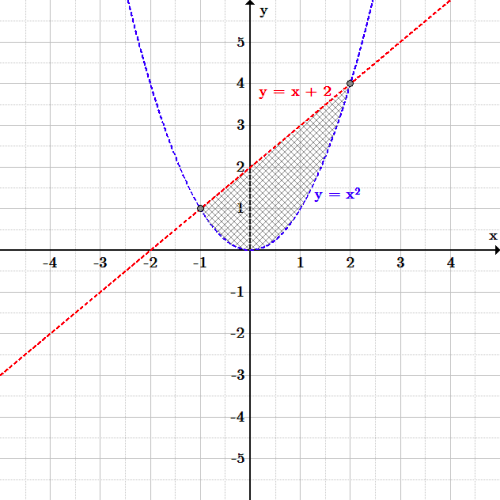## Nonlinear Systems of Equations and Problem-Solving

As with linear systems, a nonlinear system of equations (and conics) can be solved graphically and algebraically for all of its variables.

### Learning Objectives

Solve nonlinear systems of equations graphically and algebraically

### Key Takeaways

#### Key Points

• Subtracting one equation from another is an effective means for solving linear systems, but it often is difficult to use in nonlinear systems, in which the terms of two equations may be very different.
• Substitution of a variable into another equation is usually the best method for solving nonlinear systems of equations.
• Nonlinear systems of equations may have one or multiple solutions.

#### Key Terms

• system of equations: A set of formulas with multiple variables which can be solved using a specific set of values.
• conic section: Any of the four distinct shapes that are the intersections of a cone with a plane, namely the circle, ellipse, parabola, and hyperbola.
• nonlinear: An algebraic term that is raised to the power of two or higher; equivalently, a function with a curved graph.

### Conic Sections

A conic section (or just conic) is a curve obtained as the intersection of a cone (more precisely, a right circular conical surface) with a plane. In analytic geometry, a conic may be defined as a plane algebraic curve of degree 2. There are a number of other geometric definitions possible. The four types of conic section are the hyperbola, the parabola, the ellipse, and the circle, though the circle can be considered to be a special case of the ellipse.

The type of a conic corresponds to its eccentricity. Conics with eccentricity less than $1$ are ellipses, conics with eccentricity equal to $1$ are parabolas, and conics with eccentricity greater than $1$ are hyperbolas. In the focus-directrix definition of a conic, the circle is a limiting case of the ellipse with an eccentricity of $0$. In modern geometry, certain degenerate cases, such as the union of two lines, are included as conics as well.

### System of Equations

In a system of equations, two or more relationships are stated among variables. A system is solvable as long as there are as many simultaneous equations as variables. If each equation is graphed, the solution for the system can be found at the point where all the functions meet. The solution can be found either by inspection of a graph, typically by using graphing or plotting software, or algebraically.

### Nonlinear Systems

Nonlinear systems of equations, such as conic sections, include at least one equation that is nonlinear. A nonlinear equation is defined as an equation possessing at least one term that is raised to a power of 2 or more. When graphed, these equations produce curved lines.

Since at least one function has curvature, it is possible for nonlinear systems of equations to contain multiple solutions. As with linear systems of equations, substitution can be used to solve nonlinear systems for one variable and then the other.

Solving nonlinear systems of equations algebraically is similar to doing the same for linear systems of equations. However, subtraction of one equation from another can become impractical if the two equations have different terms, which is more commonly the case in nonlinear systems.

### Example

Consider, for example, the following system of equations:

\begin{align} y &= x^2 \; \qquad (1) \\ y &= x + 6 \quad (2) \end{align}Integer values of $y=x^2$ (blue) and $y=x+6$ (red): The parabola (blue) falls below the line (red) between $x=-2$ and $x=3$. For all values of $x$ less than $-2$ and greater than $3$, points on the parabola are above the line.

We can solve this system algebraically by using equation $(1)$ as a substitution. The quantity $x^2$ must be equivalent to the quantity $y$, so we substitute $x^2$ for $y$ in equation $(2)$:

\displaystyle{ \begin{align} y&=x+6 \\ x^2&=x+6 \end{align} }

This quadratic equation can be solved by moving all the equation’s components to the left before using the quadratic formula:

$x^2-x-6=0$

Using the quadratic formula, with $a = 1$, $b = -2$, and $c = -6$, it can be determined that the solutions are $x = -2$ and $x = 3$.

The solutions for $x$ can then be plugged into either of the original systems to find the value of $y$. In this example, we will use equation $(1)$:

\displaystyle{ \begin{align} y&=x^2 \\ y&=(-2)^2 \\ y&=4 \end{align} }

\displaystyle{ \begin{align} y&=x^2 \\ y&=(3)^2\\ y&=9 \end{align} }

Thus, for $x = -2$, $y = 4$, and for $x = 3$, $y = 9$.

Our final solutions are: $(-2,4)$ and $(3,9)$.

## Models Involving Nonlinear Systems of Equations

Nonlinear systems of equations can be used to solve complex problems involving multiple known relationships.

### Learning Objectives

Use nonlinear systems of equations to solve problems in the real world

### Key Takeaways

#### Key Points

• Problems involving simultaneously moving bodies can be solved using systems of equations. If at least one body accelerates or decelerates, the system is nonlinear.
• If the relationship between multiple unknown numbers is described in as many ways as there are numbers, all unknowns can be found using systems of equations. If at least one of those relationships is nonlinear, the system is nonlinear.
• Substitution is the best method for solving for simultaneous equations, although to answer a question, one may not need to solve for every variable.

#### Key Terms

• system of equations: A set of formulas with multiple variables which can be solved using a specific set of values.

Nonlinear systems of equations are not just for hypothetical discussions—they can be used to solve complex problems involving multiple known relationships.

### Real World Examples

Consider, for example, a car that begins at rest and accelerates at a constant rate of $4$ meters per second each second. Its position in meters ($y$) can be determined as a function of time in seconds ($t$), by the formula:

\displaystyle{ \begin{align} y&=\frac{1}{2}\left(4\right)t^2\\ y&=2t^2 \end{align} }

Now consider a second car, traveling at a constant speed of $20$ meters per second. Its position ($y$) in meters can be determined as a function of time ($t$) in seconds, using the following formula:

$y=20t$

When the first car begins to accelerate, the second car is $400$ meters ahead of it. To express the position of the second car relative to the first as a function of time, we can modify the second equation as such:

$y=20t+400$

To determine where the cars are when they are alongside one another and how much time has passed since the first began to accelerate, we can algebraically solve the system of equations using substitution:

\begin{align} y&=20t+400\\ 2t^2&=20t+400 \end{align}

Solving for $t$, we can find that the cars are side-by-side after $20$ seconds.

Substituting $20$ for $t$ into the equations for either of the cars, we can find that the cars meet $800$ meters ahead of the first car’s starting point. Note that a question on an exam may not prompt solutions for both variables.

Some other real-world examples of nonlinear systems include:

• Triangulation of GPS signals. A device like your cellphone receives signals from GPS satellites, which have known orbital positions around the Earth. A signal from a single satellite allows a cellphone to know that it is somewhere on a circle. Additional signals are additional circles that intersect each other, and the cellphone’s actual position is at the intersection. Three or more signals reduce the solution of the system to a single coordinate point.
• The conservation of mechanical energy can produce a system of nonlinear equations when there is an elastic (perfectly bouncy) collision. The kinetic energy of the objects depends on the speed squared, and the momentum depends on the speed directly.
• Manufacturing and design of everything, from electronic parts to metal tools to the architecture of buildings, uses computer-aided design software that helps create three-dimensional shapes from the intersection of curved lines. Rendering and visualizing these objects, and formulating a plan for constructing them, requires the software to solve nonlinear systems.

In addition to practical scenarios like the above, nonlinear systems can be used in abstract problems. For example, a question on an exam could ask:

The product of two numbers is 12, and the sum of their squares is 40. What are the numbers?

In this case, we could make an equation for each known relationship:

\begin{align} x\cdot y&=12 \\ x^2+y^2&=40 \end{align}

Substitution can be used to calculate that the numbers are 2 and 6.

## Nonlinear Systems of Inequalities

Systems of nonlinear inequalities can be solved by graphing boundary lines.

### Learning Objectives

Practice techniques for solving nonlinear systems of inequalities

### Key Takeaways

#### Key Points

• A nonlinear system of inequalities may have at least one solution; if it does, a solution may be bounded or unbounded.
• A solution for a nonlinear system of inequalities will be in a region that satisfies every inequality in the system.
• The best way to show solutions to nonlinear systems of inequalities is graphically, by shading the area that satisfies all of the system’s constituent inequalities.

#### Key Terms

• inequality: A statement that, of two quantities, one is specifically less than or greater than another. Symbols: $<$ or $\leq$ or $>$ or $\geq$, as appropriate.
• nonlinear: A polynomial expression of degree 2 or higher.
• system of equations: A set of formulas with multiple variables which can be solved using a specific set of values.

A system of inequalities consists of two or more inequalities, which are statements that one quantity is greater than or less than another. A nonlinear inequality is an inequality that involves a nonlinear expression—a polynomial function of degree 2 or higher. The most common way of solving one inequality with two variables $x$ and $y$ is to shade the region on a graph where the inequality is satisfied.Cubic function: This graph of a cubic function is an example of a nonlinear equation.

Every inequality has a boundary line, which is the equation produced by changing the inequality relation to an equals sign. The boundary line is drawn as a dashed line (if $<$ or $>$ is used) or a solid line (if $\leq$ or $\geq$ is used). One side of the boundary will have points that satisfy the inequality, and the other side will have points that falsify it. By testing individual points, the correct region can be shaded. If we have two inequalities, therefore, we shade in the overlap region, where both inequalities are simultaneously satisfied.

Consider, for example, the system including the parabolic nonlinear inequality:

$y>x^2$

and the linear inequality:

$y < x+2$

All points below the line $y=x+2$ satisfy the linear equality, and all points above the parabola $y=x^2$ satisfy the parabolic nonlinear inequality.

Graphing both inequalities reveals one region of overlap: the area where the parabola dips below the line. This area is the solution to the system.Nonlinear system of inequalities: Any point in the region between the line $y=x+2$ and the parabola $y=x^2$ satisfies the system of inequalities.

The limits of each inequality intersect at $(-1, 1)$ and $(2, 4)$. Note that the area above $y=x^2$ that is also below $y=x+2$ is closed between those two points. Whereas a solution for a linear system of equations will contain an infinite, unbounded area (lines can only pass one another a maximum of once), in many instances, a solution for a nonlinear system of equations will consist of a finite, bounded area.

This need not be the case with all nonlinear inequalities, but reversing the direction of both inequalities in the previous example would lead to an infinite solution area.